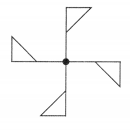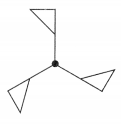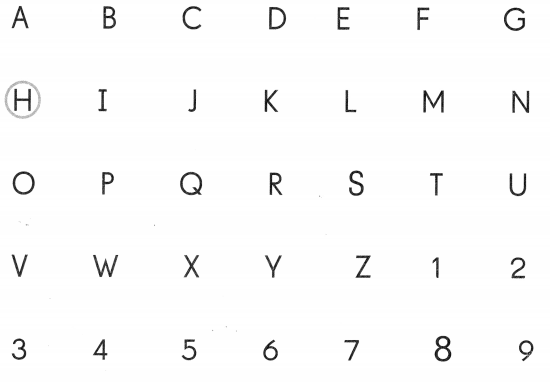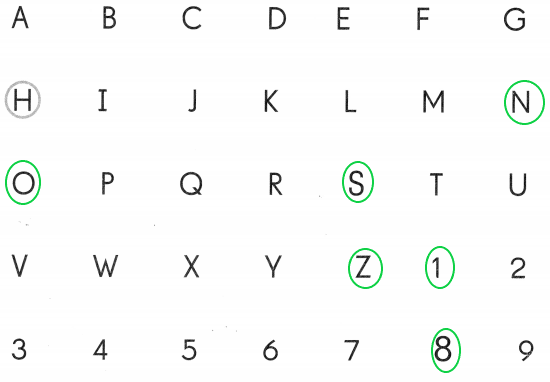# Math in Focus Grade 4 Chapter 13 Practice 2 Answer Key Rotational Symmetry

This handy Math in Focus Grade 4 Workbook Answer Key Chapter 13 Practice 2 Rotational Symmetry detailed solutions for the textbook questions.

## Math in Focus Grade 4 Chapter 13 Practice 2 Answer Key Rotational Symmetry

Decide whether each figure has rotational symmetry about the center shown. Write yes or no.

Example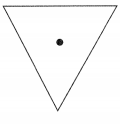Yes

Question 1.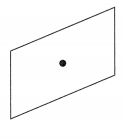Yes, The above figure has the rotational symmetry for the center shown.

Question 2.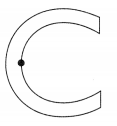No, The above figure doesn’t have the rotational symmetry for the center shown.

Question 3.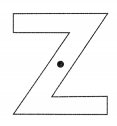Yes, The above figure has the rotational symmetry for the center shown.

Question 4.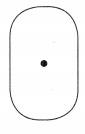Yes, The above figure has the rotational symmetry for the center shown.

Question 5.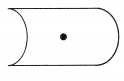No, The above figure doesn’t have the rotational symmetry for the center shown.

Decide whether each figure has rotational symmetry about the center shown. Write yes or no.

Question 6.